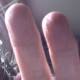# Math Monday: Passing a Cube Through Another Cube

By George Hart for the Museum of MathematicsIt is an amazing fact that it’s mathematically possible to pass a cube through a hole in another cube of the same size. If you stand a cube up on a corner (with a diagonal vertical) and shine a light down on it, its shadow is a regular hexagon. If you calculate carefully, you’ll find that a square face of the cube can fit inside this hexagon with a bit of room to spare on all sides. This means that it is possible to drill a square hole through the cube in the direction of a diagonal and the outside parts will barely connect together. Martin Raynsford has made a template for a paper model that illustrates this idea. Here is the paper cube with a square hole:You’ll need to assemble it carefully. If you look at it from the side, you see there isn’t much material to hold itself together:The image below shows one cube passing through the other.Full disclosure requires me to point out the inner cube here is actually 2% smaller than the outer cube. Martin made it this way because otherwise he found that the thin bits of remaining paper tear. But rest assured that with mathematically ideal cubes and paper, not only could the two cubes be the same size, but you can actually pass a cube through a hole in a slightly smaller cube!

More:
See all of George Hart’s Math Monday columns

## 18 thoughts on “Math Monday: Passing a Cube Through Another Cube”

1.yaya says:

Doesn’t the cube with a hole in it cease to be a cube once you cut the hole in it?

1.Blair says:

It’s talking about passing a cube through a cube, right? While thinking about that concept in your head, you can make one cube transparent hypothetically to pass the other cube through but unfortunately in reality that isn’t quite possible, so you have to make a hole in it to pass it through. Ultimately the idea is that it’s still a cube, but realistically in order to test this, a hole must be cut to pass the other cube through.

2.doctroid says:

Can you pass a hypercube through an equal size hypercube?

1.Sam Bleckley says:

I’m pretty sure a hypercube exactly cuts itself; the largest cross-section of a unit hypercube is an octahedron (sqrt(2) on a side) and the smallest cross section is a unit cube. A hole that would fit the cube into the octahedron would slice the octahedron into 6 pyramids.

1.Sam Bleckley says:

Sorry, I lie. The way I did it makes two pyramids and an odd doughnut, but I’m not positive I found the best way to pack the cube in.

3.gerd says:

Nice….
i find you should colour the one cube different to the other parts of the second cube.

4.John Bramfeld says:

The ratio between a cube and the maximum size of the cube that can fit through it would be an interesting number.

5. Demostración visual de la paradoja del cubo de Ruperto - Gaussianos | Gaussianos says:

[…] pues ahora el gran George Hart nos trae, a través de Math Monday, una especie de maqueta para realizar la comprobación de este paradoja de la intuición, pero […]

6.Miroslava von Schlockbaum says:

Somehow the lede got buried here. This is essentially “Prince Rupert’s Cube Paradox” and the paradoxical part is that the cube that can be passed is (slightly) *larger* than the cube with the hole in it. (only about ~6% larger, but hey… it’s worth pointing that out)